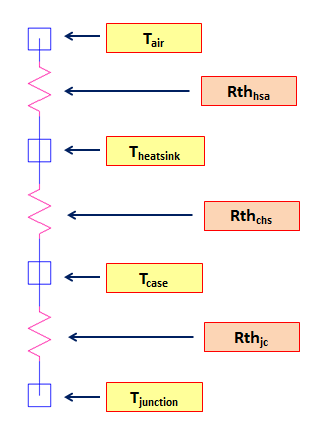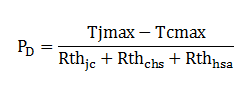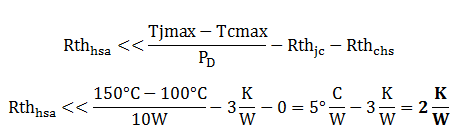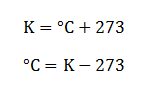# Heat Sink Thermal Resistance Calculation Easy Explanation

For power devices like power MOSFETs, power BJT and power diodes, heat sink is very important to provide thermal relief. From the name itself, it will sink the heat from the power devices and help controls the temperature in the safe zone. In this article I will discuss heat sink thermal resistance calculation. For a system with forced cooling method, heat sink size may not that big. However, for convection cooling or natural cooling the heat sink size will really matters. This heat sink thermal resistance calculation is being prepared especially for convection cooling.

In forced cooling system like the one with fan, a small heat sink size will be enough. There will be no need for detailed analysis normally on how exactly the size of the heat sink is. This will not be the case for convection or natural cooling. The heat sink’s effectiveness is being measured in terms of its thermal resistance. There will be physically big-looking heat sink but has higher thermal resistance than a small-looking heat sink. By the way, an ideal heat sink has zero thermal resistance. In real application, a smaller thermal resistance heat sink is preferred.

## Important Explanations Needed for Heat Sink Thermal Resistance Calculation

Below illustration is very important for heat sink thermal resistance calculation. The figure itself explains everything so no need to elaborate.In between temperatures, there is a thermal resistance as illustrated below.Below is an equation relating the temperatures and thermal resistances.The heat sink thermal resistance and what we are after of is the variable Rthhsa. By simple re-arrangement of the above equation will give the Rthhsa.

In this article we are not going to design heat sinks nor measure its thermal resistance. We are going to calculate what size of heat sink our circuit needs instead. There are many off the shelf heat sinks available online wherein you can select from. For instance the circuit needs a heat sink with 1’C/W thermal resistance; buy a heat sink with thermal resistance lower than this.

## Sample Heat Sink Thermal Resistance Calculation

A power MOSFET has a total computed power dissipation of 10 watts. The device maximum junction temperature is 150’C. The actual case temperature of the MOSFET must be maintained equal to or below 100’C for a reliable operation. The MOSFET thermal resistance from junction to case is 3 K/W. Determine the minimum heat sink size needed.

#### Solution

Rthjc is specified above as 3 K/W. Rthchs is the thermal resistance between the MOSFET case and the heat sink. Basically this is the thermal resistance of the element that binds the case and the heat sink. This can be an LMS, a screw, a bolt, a clip and so on. In recent SMPS, LMS is popular since it will not consume space. In this heat sink thermal resistance calculation example, the thermal resistance between MOSFET case and the heat sink is not provided. We can just neglect this one considering that the MOSFET body is perfectly binds with the heat sink. However, we should consider a margin so that we can accommodate this assumption. We will use the given equation above as re-writen below.What we are after of is the term Rthhsa which is the thermal resistance of the heat sink. Modifying the above equation we getRememeber that we are neglecting the thermal resistance from MOSFET case to heat sink; you may use a heat sink with thermal resistance that is very much lower than 2 K/W.

## Understanding Kelvin and Degrees Celsius Notation

In the above example, the junction and the case

temperatures are expressed in terms of degrees Celsius. On the other hand the thermal resistance from junction to case (Rthjc) has a unit of K/W.

How come that 5’C/W – 3K/W = 2K/W? We can only directly do subtraction if the units are the same.

Actually, there is nothing wrong on the above computations. The thermal resistance from junction to ambient or junction to case is specified in the datasheets in either ‘C/W or K/W. They are the same and no need for unit conversion.

### This is the explanation…When we add a constant 273 on the temperature in degrees Celsius, it will become already a Kelvin.

Using the same data in the above heat sink thermal resistance calculation sample and we will apply the above conversion from ‘C to K…The term inside the close and open parenthesis is already in kelvin. If we further simplify the equation, the constant 273 will be cancelled out and what will remain is the term (150-100) which is in unit kelvin now divided by 10W. Subtracted by 3 K/W, the result is 2 K/W. Therefore, don’t mind to do unit conversion.